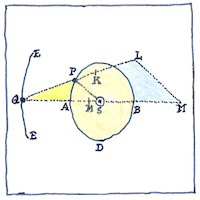# 1687 Principia Mathematica

Tom Sharp

## Principia Mathematica

By 1675, Isaac Newton had worked out the principles of planetary orbits—bodies moving freely through space subject to only gravity and their own inertia— how the planet must be balanced between centrifugal force and the gravity of the sun acting at a distance, and how its orbit must be elliptical with the sun at one focus— but Isaac hadn’t wanted to be brought down to earth— balanced between his sensitivity to criticism and his friend Edmond Halley coaxing him to publish.

## Mathematical modeling

Almost invariably, physics today is expressed using mathematical models, which Newton, in Principia Mathematica, first established as a model, going so far as to develop a new mathematical method—his infinitesimal calculus. Thus I claim M = Ie (meaning is explanation of interest) and I = Σ Sd + Ds (interest is the recognition of hidden differences between similar things and hidden similarities between different things).

## Universal gravitation

That all things in the universe great and small attract each other with force proportional to the product of their masses and inversely proportional to the square of their distance was irrefutably true and obvious until Einstein taught in his theory of general relativity that gravity was not mutual attraction but an attribute of the curvature of space and time leads to the theory that the distortion of the universe perceived while under the influence of LSD is no less valid than the distortion perceived while under the influence of mild tea so we are universally attracted to ideas great and small according to how far apart we are from them.

Isaac Newton, in publishing the Principia, started a revolution in physics. The book is important not just for the physical laws that it established, but for its style and its mathematical methods. In addition to inventing infinitesimal calculus, establishing the laws of gravitation and motion, Newton invented the reflecting telescope, and discovered that white light is composed of a spectrum of colors.

Newton was a great genius and many consider him the most influential scientist of all time. (Others think of Einstein.)